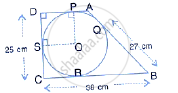Share

In the Following Figure, a Circle is Inscribed in the Quadrilateral Abcd. If Bc = 38 Cm, Qb = 27 Cm, Dc = 25 Cm and that Ad is Perpendicular to Dc, Find the Radius of the Circle. - ICSE Class 10 - Mathematics

ConceptChord Properties - the Perpendicular to a Chord from the Center Bisects the Chord (Without Proof)

Question

In the following figure, a circle is inscribed in the quadrilateral ABCD.If BC = 38 cm, QB = 27 cm, DC = 25 cm and that AD is perpendicular to DC, find the radius of the circle.

Solution

From the figure we see that BQ = BR = 27 cm (since length of the tangent segments from an external point are equal)
As BC = 38 cm
⇒ CR = CB − BR = 38 − 27
= 11 cm
Again,
CR = CS = 11cm (length of tangent segments from an external point are equal)
Now, as DC = 25 cm
∴ DS = DC − SC
= 25 − 11
= 14 cm
Now, in quadrilateral DSOP,
∠PDS = 90° (given)
∠ OSD = 90°, ∠OPD = 90° (since tangent is perpendicular to the
radius through the point of contact)
⇒ DSOP is a parallelogram
⇒ OP ∥ SD and  ⇒ PD ∥ OS
Now, as OP = OS (radii of the same circle)
⇒ OPDS is a square. ∴ DS = OP = 14cm
∴ radius of the circle = 14 cm

Is there an error in this question or solution?

APPEARS IN

Solution In the Following Figure, a Circle is Inscribed in the Quadrilateral Abcd. If Bc = 38 Cm, Qb = 27 Cm, Dc = 25 Cm and that Ad is Perpendicular to Dc, Find the Radius of the Circle. Concept: Chord Properties - the Perpendicular to a Chord from the Center Bisects the Chord (Without Proof).
S